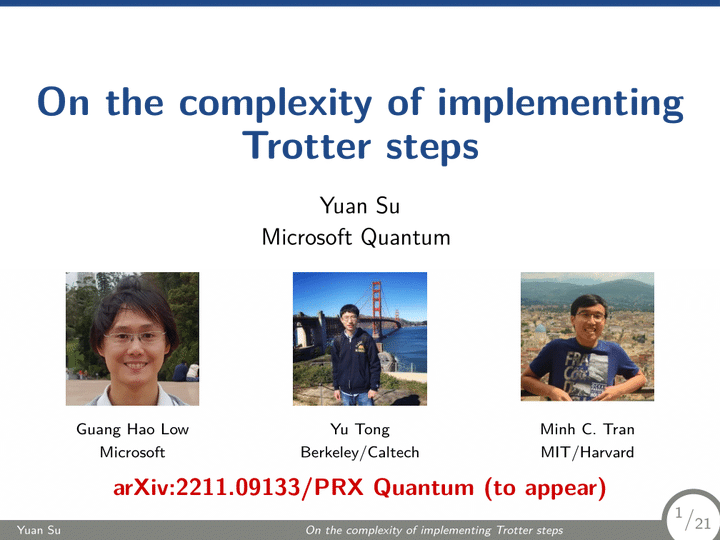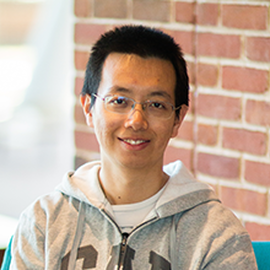# On the complexity of implementing Trotter steps (IOP-CAS 2023)### Abstract

Quantum dynamics can be simulated on a quantum computer by exponentiating elementary terms from the Hamiltonian in a sequential manner. However, such an implementation of Trotter steps has gate complexity depending on the total Hamiltonian term number, comparing unfavorably to algorithms using more advanced techniques. We develop methods to perform faster Trotter steps with complexity sublinear in the number of terms. We achieve this for a class of Hamiltonians whose interaction strength decays with distance according to power law. Our methods include one based on a recursive block encoding and one based on an average-cost simulation, overcoming the normalization-factor barrier of these advanced quantum simulation techniques. We also realize faster Trotter steps when certain blocks of Hamiltonian coefficients have low rank. Combining with a tighter error analysis, we show that it suffices to use $\left(\eta^{1/3}n^{1/3}+\frac{n^{2/3}}{\eta^{2/3}}\right)n^{1+o(1)}$ gates to simulate uniform electron gas with $n$ spin orbitals and $\eta$ electrons in second quantization in real space, asymptotically improving over the best previous work. We obtain an analogous result when the external potential of nuclei is introduced under the Born-Oppenheimer approximation. We prove a circuit lower bound when the Hamiltonian coefficients take a continuum range of values, showing that generic $n$-qubit $2$-local Hamiltonians with commuting terms require at least $\Omega(n^2)$ gates to evolve with accuracy $\epsilon=\Omega(1/poly(n))$ for time $t=\Omega(\epsilon)$. Our proof is based on a gate-efficient reduction from the approximate synthesis of diagonal unitaries within the Hamming weight-$2$ subspace, which may be of independent interest. Our result thus suggests the use of Hamiltonian structural properties as both necessary and sufficient to implement Trotter steps with lower gate complexity.

Date
Apr 13, 2023
Location
Institute of Physics, Chinese Academy of Sciences, Beijing 100190, China (online event)##### Yuan Su
###### Senior Researcher

I work on quantum algorithms for simulating Hamiltonian dynamics. I am particularly interested in the design, analysis, implementation, and application of quantum simulation.